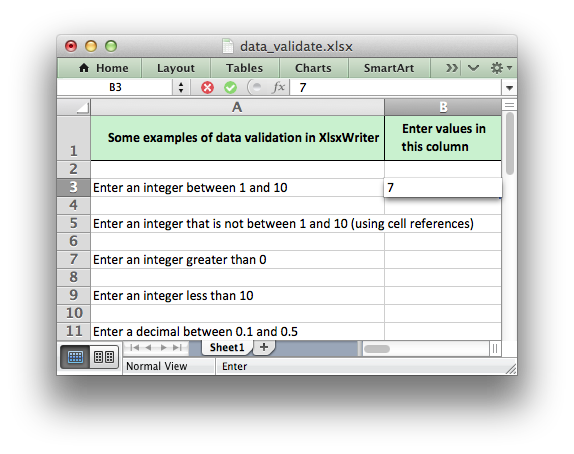# Example: Data Validation and Drop Down Lists

Example of how to add data validation and drop down lists to an XlsxWriter file. Data validation is a way of limiting user input to certain ranges or to allow a selection from a drop down list.```###############################################################################
#
# Example of how to add data validation and dropdown lists to an
# XlsxWriter file.
#
# Data validation is a feature of Excel which allows you to restrict
# the data that a user enters in a cell and to display help and
# warning messages. It also allows you to restrict input to values in
# a drop down list.
#
# Copyright 2013-2023, John McNamara, jmcnamara@cpan.org
#
from datetime import date, time
import xlsxwriter

workbook = xlsxwriter.Workbook("data_validate.xlsx")

{
"border": 1,
"bg_color": "#C6EFCE",
"bold": True,
"text_wrap": True,
"valign": "vcenter",
"indent": 1,
}
)

# Set up layout of the worksheet.
worksheet.set_column("A:A", 68)
worksheet.set_column("B:B", 15)
worksheet.set_column("D:D", 15)
worksheet.set_row(0, 36)

# Write the header cells and some data that will be used in the examples.
heading1 = "Some examples of data validation in XlsxWriter"
heading2 = "Enter values in this column"

worksheet.write_row("D3", ["Integers", 1, 10])
worksheet.write_row("D4", ["List data", "open", "high", "close"])
worksheet.write_row("D5", ["Formula", "=AND(F5=50,G5=60)", 50, 60])

# Example 1. Limiting input to an integer in a fixed range.
#
txt = "Enter an integer between 1 and 10"

worksheet.write("A3", txt)
worksheet.data_validation(
"B3", {"validate": "integer", "criteria": "between", "minimum": 1, "maximum": 10}
)

# Example 2. Limiting input to an integer outside a fixed range.
#
txt = "Enter an integer that is not between 1 and 10 (using cell references)"

worksheet.write("A5", txt)
worksheet.data_validation(
"B5",
{
"validate": "integer",
"criteria": "not between",
"minimum": "=E3",
"maximum": "=F3",
},
)

# Example 3. Limiting input to an integer greater than a fixed value.
#
txt = "Enter an integer greater than 0"

worksheet.write("A7", txt)
worksheet.data_validation("B7", {"validate": "integer", "criteria": ">", "value": 0})

# Example 4. Limiting input to an integer less than a fixed value.
#
txt = "Enter an integer less than 10"

worksheet.write("A9", txt)
worksheet.data_validation("B9", {"validate": "integer", "criteria": "<", "value": 10})

# Example 5. Limiting input to a decimal in a fixed range.
#
txt = "Enter a decimal between 0.1 and 0.5"

worksheet.write("A11", txt)
worksheet.data_validation(
"B11",
{"validate": "decimal", "criteria": "between", "minimum": 0.1, "maximum": 0.5},
)

# Example 6. Limiting input to a value in a dropdown list.
#
txt = "Select a value from a drop down list"

worksheet.write("A13", txt)
worksheet.data_validation(
"B13", {"validate": "list", "source": ["open", "high", "close"]}
)

# Example 7. Limiting input to a value in a dropdown list.
#
txt = "Select a value from a drop down list (using a cell range)"

worksheet.write("A15", txt)
worksheet.data_validation("B15", {"validate": "list", "source": "=\$E\$4:\$G\$4"})

# Example 8. Limiting input to a date in a fixed range.
#
txt = "Enter a date between 1/1/2013 and 12/12/2013"

worksheet.write("A17", txt)
worksheet.data_validation(
"B17",
{
"validate": "date",
"criteria": "between",
"minimum": date(2013, 1, 1),
"maximum": date(2013, 12, 12),
},
)

# Example 9. Limiting input to a time in a fixed range.
#
txt = "Enter a time between 6:00 and 12:00"

worksheet.write("A19", txt)
worksheet.data_validation(
"B19",
{
"validate": "time",
"criteria": "between",
"minimum": time(6, 0),
"maximum": time(12, 0),
},
)

# Example 10. Limiting input to a string greater than a fixed length.
#
txt = "Enter a string longer than 3 characters"

worksheet.write("A21", txt)
worksheet.data_validation("B21", {"validate": "length", "criteria": ">", "value": 3})

# Example 11. Limiting input based on a formula.
#
txt = 'Enter a value if the following is true "=AND(F5=50,G5=60)"'

worksheet.write("A23", txt)
worksheet.data_validation("B23", {"validate": "custom", "value": "=AND(F5=50,G5=60)"})

# Example 12. Displaying and modifying data validation messages.
#
txt = "Displays a message when you select the cell"

worksheet.write("A25", txt)
worksheet.data_validation(
"B25",
{
"validate": "integer",
"criteria": "between",
"minimum": 1,
"maximum": 100,
"input_title": "Enter an integer:",
"input_message": "between 1 and 100",
},
)

# Example 13. Displaying and modifying data validation messages.
#
txt = "Display a custom error message when integer isn't between 1 and 100"

worksheet.write("A27", txt)
worksheet.data_validation(
"B27",
{
"validate": "integer",
"criteria": "between",
"minimum": 1,
"maximum": 100,
"input_title": "Enter an integer:",
"input_message": "between 1 and 100",
"error_title": "Input value is not valid!",
"error_message": "It should be an integer between 1 and 100",
},
)

# Example 14. Displaying and modifying data validation messages.
#
txt = "Display a custom info message when integer isn't between 1 and 100"

worksheet.write("A29", txt)
worksheet.data_validation(
"B29",
{
"validate": "integer",
"criteria": "between",
"minimum": 1,
"maximum": 100,
"input_title": "Enter an integer:",
"input_message": "between 1 and 100",
"error_title": "Input value is not valid!",
"error_message": "It should be an integer between 1 and 100",
"error_type": "information",
},
)

workbook.close()
```